# TD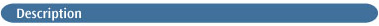This method keyword requests an excited state energy calculation using the time-dependent Hartree-Fock or DFT method [109,110,111].

Note that the normalization criteria used is <X+Y|X-Y>=1.

Electronic circular dichroism (ECD) analysis is also performed during these calculations [255,256,257,258,259,260]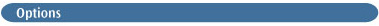Singlets
Solve only for singlet excited states. Only effective for closed-shell systems, for which it is the default.

Triplets
Solve only for triplet excited states. Only effective for closed-shell systems.

50-50
Solve for half triplet and half singlet states. Only effective for closed-shell systems.

Root=N
Specifies the state of interest. The default is the first excited state (N=1).

NStates=M
Solve for M states (the default is 3). If 50-50 is requested, NStates gives the number of each type of state for which to solve (i.e., the default is 3 singlets and 3 triplets).

Add=N
Read converged states off the checkpoint file and solve for an additional N states. This option implies Read as well.

Read
Reads initial guesses for the states off the checkpoint file. Note that, unlike for SCF, an initial guess for one basis set cannot be used for a different one.

EqSolv
Whether to perform equilibrium or non-equilibrium PCM solvation. NonEqSolv is the default.

IVOGuess
Force use of IVO guess. This is the default for TD Hartree-Fock. NoIVOGuess forces the use of canonical single excitations for guess, and it is the default for TD-DFT. The HFIVOGuess option forces the use of Hartree-Fock IVOs for the guess, even for TD-DFT.

SOS
Do sum-over states polarizabilities, etc. By default, all excited states are solved for. A list of frequencies at which to do the sums is read in. Zero frequency is always done and need not be in the list.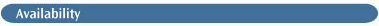Energies using Hartree-Fock or a DFT method. Optimizations are available using numerical gradients.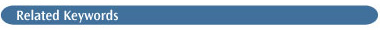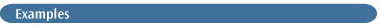Here is the key part of the output from a TD excited states calculation:

```Excitation energies and oscillator strengths:

Excited State   1:   Singlet-A2    4.1280 eV   300.35 nm   f=0.0000
8 ->  9         0.68197
This state for optimization and/or second-order correction.
Copying the excited state density for this state as the 1-particle
RhoCI density.

Excited State   2:   Singlet-B2    6.4912 eV   191.00 nm   f=0.0356
8 ->  10        0.70318

Excited State   3:   Singlet-A1    7.4378 eV   166.69 nm   f=0.0541
8 ->  11        0.70219 ```

The results on each state are summarized, including the spin and spatial symmetry, the excitation energy, the oscillator strength, and (on the second line for each state) the largest coefficients in the CI expansion.

The ECD results appear in the output as follows:

```<0|del|b> * <b|rxdel|0>  (Au), Rotatory Strengths (R) in
cgs (10**-40 erg-esu-cm/Gauss)
state          X            Y            Z     R(velocity)
1         0.0045      -0.0007      -0.0001     5.6444
2        -0.0040      -0.0004       0.0018    -2.9442
3        -0.0007      -0.0024       0.0043     1.3201
<0|r|b> * <b|rxdel|0>  (Au), Rotatory Strengths (R) in
cgs (10**-40 erg-esu-cm/Gauss)
state          X            Y            Z     R(length)
1        -0.0300       0.0048       0.0007     5.7826
2         0.0193       0.0017      -0.0083    -3.0068
3         0.0034       0.0111      -0.0200     1.3067 ```